### Home > INT3 > Chapter 12 > Lesson 12.1.2 > Problem12-24

12-24.

Jeremy uses the $\sin^{-1}$ button on his calculator to solve a trigonometric equation and gets the solution $37^\circ$. He knows that there must be more than one solution. What are the rest of the solutions to Jeremy’s equation? Use a unit circle to explain how you determined all of the solutions.

Using a unit circle:

Draw a Unit circle and estimate $37$ degrees.

Circle, with horizontal diameter, & radius to point in the first quadrant on the circle, with central angle labeled, 37 degrees.

Since sin is height, draw in the height to the $x$-axis.

Added to diagram, vertical segment from point in first quadrant on circle, perpendicular to x axis.

Find and draw the same height any other place it may occur on the unit circle.

Added to diagram, additional vertical segment, of same height, reflected over the, y axis, in the second quadrant.

Calculate the new angles.

$180^\circ − 37^\circ = 143^\circ$, so $37 + 2πn$ and $143 + 2πn$ where $n$ is any integer.

Added to diagram, segment from point in second quadrant on circle to center, with angle from positive x axis, to the new segment, labeled 143 degrees.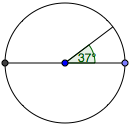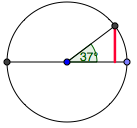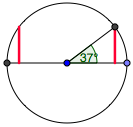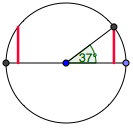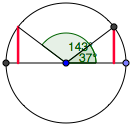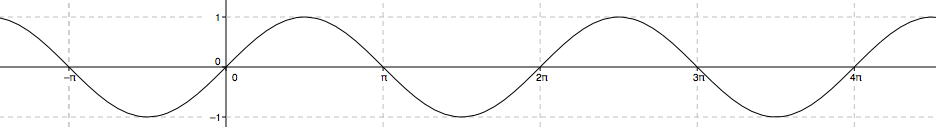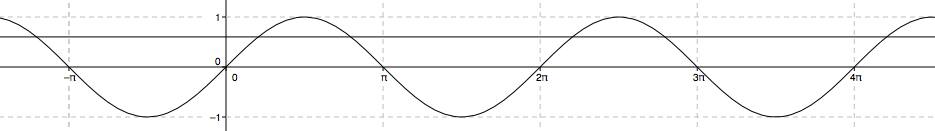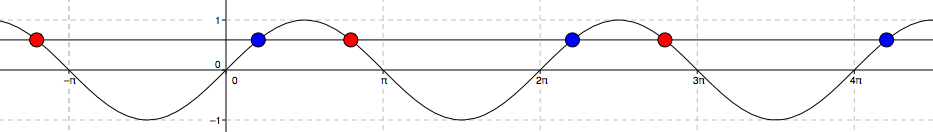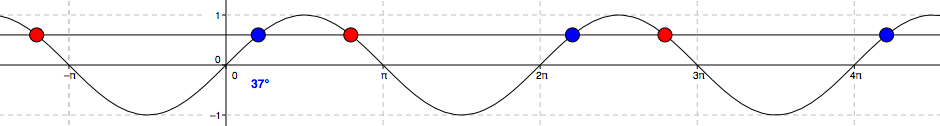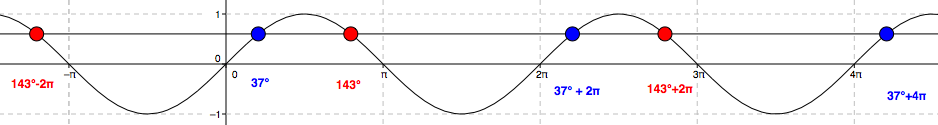Using a graph:

Draw the sine curve.

Repeating wave curve, first visible low & high points: (negative pi halves, comma negative 1) & (pi halves, comma 1), continuing in that pattern, just past 4 pi.

Draw $y=\sin(37)$

Added to graph, horizontal line at approximately, y = 0.6.

Find the $x$-values where the two graphs intersect.

Added to graph, red points at the intersections of the horizontal line with the wave curve, left of negative pi, pi, & 3 pi. Blue points at the intersections, right of, y axis, 2 pi, & 4 pi.

Label the solution $37^\circ$.

Label added to first blue point, right of, y axis, 37 degrees.

Use Symmetry to find other solutions.

Labels added to red points, from left to right, 143 degrees minus 2 pi, 143 degrees, 143 degrees + 2 pi. Labels added to other blue points, from left to right, 37 degrees + 2 pi, 37 degrees + 4 pi.